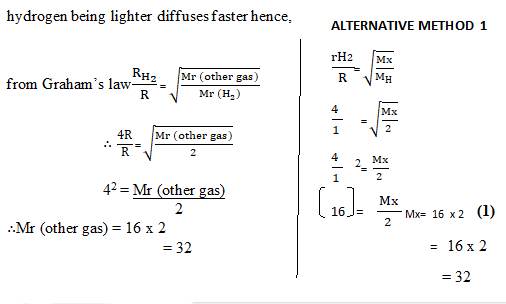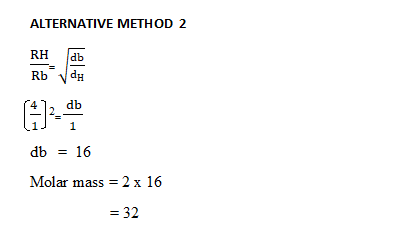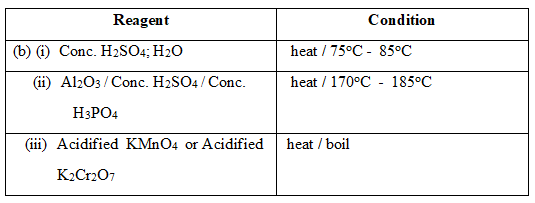### Question 2

1. The relative rates of diffusion of two gases is 1:4, if the lighter gas is hydrogen.

Calculate the relative molecular mass of the other gas.[H2 = 2.00]

2. State why the relative atomic mass of some elements is not a whole number.

[5 marks]

1. State the reagents and conditions necessary for each of the following conversions:

1. CH2 = CH2 → CH3 CH2 OH.

2. CH3CH2OH → CH2 = CH2.

3. CH3 CH2 OH → CH3COOH.

[6 marks]

1. State Le Chatelier’s principle.

2. Consider the following reaction: 2SO2(g) + O2(g) ⇌ 2SO3 (g); 𝛥H < O
Deduce, giving reasons, the conditions for maximum yield of SO3(g) if the reaction is exothermic in the forward direction.

[6 marks]

1. State two factors which determine atomic radius.

2. State:
1. one similarity;

2. two differences between a Is orbital and a 2s orbital.
3. [6 marks]

### Observation

This was not a popular question as majority of the candidates avoided it. The performance of candidates in the question was average.

In part (a)candidates could not use the principle of Graham’s law to solve the question.

In part (b) the reagents and the conditions necessary for the conversion of the organic compounds were not correctly stated.

In part (c) A fair attempt was made at stating and applying Le Chatelier’s principle in deducing the conditions for maximum yield of SO3 from the reaction equation.

In part (d) majority of the candidates stated the differences between a 1s orbital and a 2s orbital.

1. .2. because they are weighted averages of isotopic masses

Accept because of the existence of isotopes

1. .

2.1. When a stress / external constraint is applied on a system in chemical equilibrium, the equilibrium position shifts in the direction that will cancel / nullify the stress / external constraint.

2.  Conditions Reasons 1 High pressure Forward reaction proceeds with decrease in number of moles of gas/ volume of gas 2 Low temperature Suitable catalyst Because reaction is exothermic catalyst takes care of the equilibrium 3 Excess of oxygen/ K2Cr2O Equilibrium shifts to the right Excess of SO2/ Removal of SO3

• nuclear charge

• number of electron shells

• each can accommodate a maximum of only two electrons

• same shape/spherical shape.

• 1s is in the first main shell / n = 1 while

• 2s is in the second main shell / n = 2

• 1s has a lower energy than 2s

• 2s is larger in size than 1s